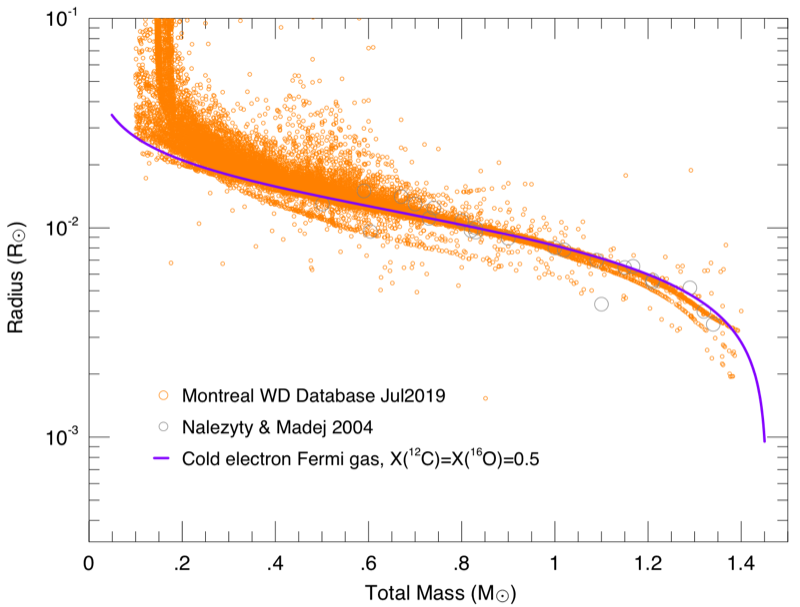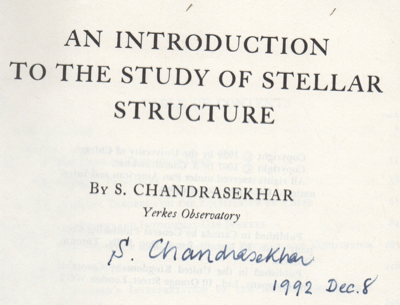Cococubed.com Cold White Dwarfs

Home

Astronomy research
Software instruments
Stellar equation of states
EOS with ionization
EOS for supernovae
Chemical potentials
Stellar atmospheres

Voigt Function
Jeans escape
Polytropic stars
Cold white dwarfs

Cold neutron stars
Stellar opacities
Neutrino energy loss rates
Ephemeris routines
Fermi-Dirac functions

Polyhedra volume
Plane - cube intersection
Coating an ellipsoid

Nuclear reaction networks
Nuclear statistical equilibrium
Laminar deflagrations
CJ detonations
ZND detonations

Fitting to conic sections
Unusual linear algebra
Derivatives on uneven grids

Supernova light curves
Exact Riemann solutions
1D PPM hydrodynamics
Hydrodynamic test cases
Galactic chemical evolution

Universal two-body problem
Circular and elliptical 3 body
The pendulum
Phyllotaxis

MESA
MESA-Web
FLASH

Zingale's software
Brown's dStar
GR1D code
Herwig's NuGRID
Meyer's NetNuc
Presentations
Illustrations
Public Outreach
Education materials
2022 ASU Solar Systems Astronomy
2022 ASU Energy in Everyday Life

AAS Journals
2022 Earendel, A Highly Magnified Star
2022 TV Columbae, Micronova
2022 White Dwarfs and 12C(α,γ)16O
2022 MESA in Don't Look Up
2022 MESA Marketplace
2022 MESA Summer School
2022 MESA Classroom
2021 Bill Paxton, Tinsley Prize

Contact: F.X.Timmes
my one page vitae,
full vitae,
research statement, and
teaching statement.

The tool in coldwd.tbz generates models of stars in hydrostatic equilibrium with a cold electron Fermi gas equation of state: \begin{equation} \begin{split} x & = \left [ \dfrac{3}{8 \pi} \left ( \dfrac{h}{m_ec}\right )^3 N_A Y_e \rho \right ]^{1/3} \\ f(x) & = x (x^2 + 1)^{1/2}(2x^2 - 3) + 3\ln(x + (x^2 + 1)^{1/2}) \\ g(x) & = 8x^3 \left [ (x^2 + 1)^{1/2} -1) \right ] - f(x) \\ P_e & = \dfrac{\pi m_e^4 c^5}{3 h^3} \cdot f(x) \hskip 1.0in E_e = \dfrac{\pi m_e^4 c^5}{3 h^3} \cdot g(x) \end{split} \label{eq1} \tag{1} \end{equation} The derivatives of the pressure and energy with respct to the density are also returned by the equation of state module. A general relativistic Tolman-Oppenheimer-Volkoff (TOV) correction to the equation for hydrostatic equilibrium is avaliable as an option. A quote from Icko about generating white dwarf models comes to mind ...

The equations above suffer a loss of numerical precision for x ≪ 1 due to the subtraction of two nearly equal terms. These expansions are used instead \begin{equation} \begin{split} f(x) & = \frac{8}{5} x^5 - \frac{4}{7} x^7 + \frac{1}{3} x^9 - \frac{5}{22} x^{11} + \frac{35}{208} x^{13} - \frac{21}{160} x^{15} + \frac{231}{2176} x^{17} + \mathcal{O}(x^{19}) \\ g(x) & = \frac{12}{5} x^5 - \frac{3}{7} x^7 + \frac{1}{6} x^9 - \frac{15}{176} x^{11} + \frac{21}{416} x^{13} - \frac{21}{640} x^{15} + \frac{99}{4352} x^{17} + \mathcal{O}(x^{19}) \ . \end{split} \label{eq2} \tag{2} \end{equation}
The first plot below shows the central density vs mass relationship between a cold electron Fermi gas equation of state and a polytropic equation of state.

A cold electron Fermi gas at low central densities (x ≪ 1) approaches the well-known nonrelativistic form $P = 1.004 \times 10^{13} \ (Y_e \rho)^{5/3} \ {\rm erg} \ {\rm cm}^{-3}$, as can be seen by the leading order $x^5$ series expansion term for f(x) above. In this limit the electrons are well approximated by a n = 3/2, γ = 1 + 1 /n = 5/3 polytropic equation of state.

A cold electron Fermi gas at high central densities (x ≫ 1) approaches the relativistic form $P = 1.2435 \times 10^{15} \ (Y_e \rho)^{4/3} \ {\rm erg} \ {\rm cm}^{-3}$; expansions in this limit are in the source code for reference but are not used as they are not needed. In this limit the electrons are well approximated by a n = 3 γ = 1 + 1 /n = 4/3 polytropic equation of state – the celebrated Chandrasekhar limit.It was a good day. Chicago. 2nd floor LASR. One in an impeccable brown suit and the other in blue overalls, white t-shirt, and Sear's DieHard steel-toe black shoes.

Please cite the relevant references if you publish a piece of work that use these codes, pieces of these codes, or modified versions of them. Offer co-authorship as appropriate.